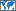## BODMAS

 Definition : Brackets, Orders, Division, Multiplication, Addition, Subtraction Category : Academic & Science » Mathematics Country/Region : WorldwidePopularity : Type : Mnemonic

# What does BODMAS mean?

BODMAS stands for Brackets, Orders, Division, Multiplication, Addition, Subtraction. BODMAS is a rule used to execute operations in a given mathematical expression. It is a mnemonic which can be used to remember the order in which to solve a mathematical problem. According to the BODMAS rule: Brackets first, followed by Orders (powers and roots), then division, multiplication, addition, and subtraction from left to right. Brackets mean the parts of a calculation inside brackets always come first. Orders mean the numbers involving powers or square roots. Solving the problem in the wrong order will result in a wrong answer.

In the United States, the term PEMDAS is commonly used, which stands for Parentheses, Exponents, Multiplication/Division, Addition/Subtraction.

In Canada and New Zealand, the term BEDMAS is commonly used, which stands for Brackets, Exponents, Division/Multiplication, Addition/Subtraction.

In the United Kingdom, the term BIDMAS is commonly used, which stands for Brackets, Indices, Division/Multiplication, Addition/Subtraction.